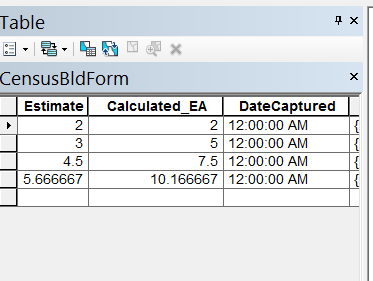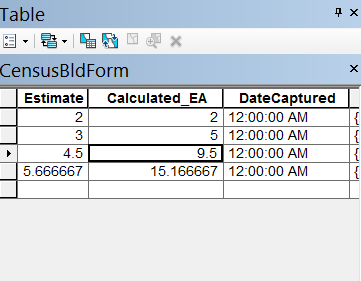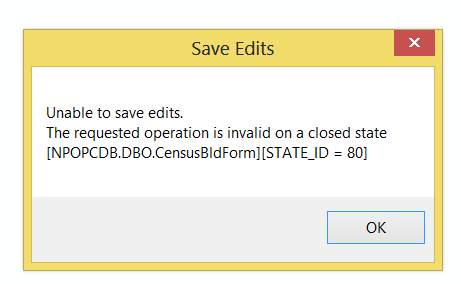# My python script calculates only the value of the immediate previous record

2009
10
04-26-2016 11:26 AMOccasional Contributor II

My python script calculates only the value of the immediate previous record instead of calculating the values of all preceding records before that particular record. The example (the image) below is the undesired output;While the image below here is what i want to achieve;This is my python script i used;

```import arcpy
from arcpy import da
CensusBldForm = "CensusBldForm"
Calculated_EA1=0
with arcpy.da.UpdateCursor(CensusBldForm, ["OBJECTID", "EstimatedPopulationinEA", "Calculated_EA"]) as cursor:
for row in cursor:
Calculated_EA2 = row
row = (Calculated_EA1 + Calculated_EA2)
Calculated_EA1 = Calculated_EA2
cursor.updateRow(row)```

Kindly assist me with the above please.

Tags (1)
1 Solution

Accepted SolutionsRegular Contributor III

Change this line

Calculated_EA1 = Calculated_EA2

To this

Calculated_EA1 += Calculated_EA2

10 RepliesRegular Contributor III

Change this line

Calculated_EA1 = Calculated_EA2

To this

Calculated_EA1 += Calculated_EA2Occasional Contributor II

Thanks, it worked like magicRegular Contributor IIIOccasional Contributor II

My edits don't get saved after running the script, because i have to start an edit session before running the script;

CensusBldForm = "CensusBldForm"

Calculated_EA1=0

with arcpy.da.UpdateCursor(CensusBldForm, ["OBJECTID", "EstimatedPopulationinEA", "Calculated_EA"]) as cursor:

for row in cursor:

Calculated_EA2 = row

row = (Calculated_EA1 + Calculated_EA2)

Calculated_EA1 += Calculated_EA2

cursor.updateRow(row)

What do you think might be wrong or better still can i get it to run without starting an edit session i.e using a arcpy.UpdateCursor instead of arcpy.da.UpdateCursor? ThanksOccasional Contributor II

This is the error message i get when i want to save edits;byMVP Esteemed Contributor

a wild guess that you aren't doing this on a file stored on your local machine but from sde or some other thing like that.  That error message has a history... there are some suggestion which you can search on geonet and narrow down the issue.  This is just one of severalOccasional Contributor II

Thanks, i'm actually running my script against an SDE GeodatabaseOccasional Contributor II

Thanks i changed from the default version to another version and i didn't get any error and my edits where retained as well.byMVP Esteemed Contributor